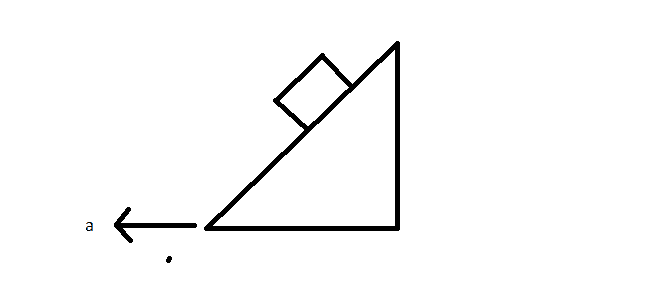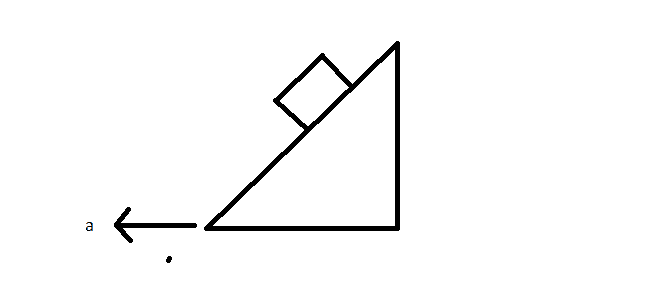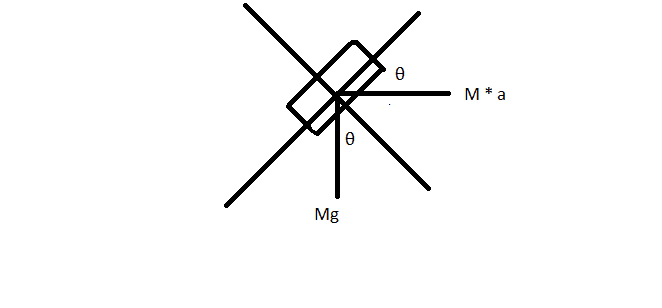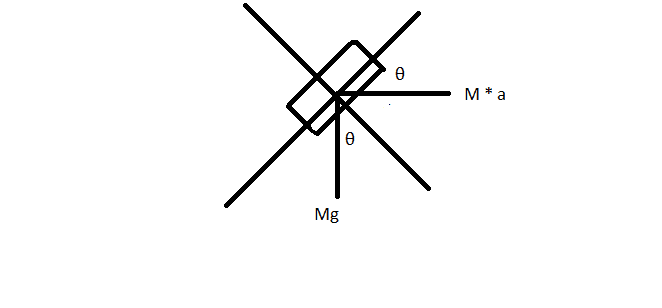# Normal forces question

• B
Biker
In the past I used to understand it in some kind of way. But it doesn't make a lot of sense sometimes.

In high school, we usually take examples where the object is placed in an incline and the Normal force is equal to mg cos theta where theta is the angle of the incline. It should equal to that because it doesn't mention anything about vertical acceleration if we use the standard axes.

However, I try to solve a bit more complex problems such as wedges and stuff. I usually treat normal force as a force that changes and becomes the way that I want it to be.

As for example, If an object is placed on an incline and the incline (faces the left) has an acceleration to the left. We usually say that the normal force is bigger in magnitude than gravity because I believe that the incline and the object are much more compressed so there is more repel forces between atoms. What really bothers me is how do we ensure that the normal force will be like that in real life? It might be a bit confusing, but If you see it from my point of view you might know what I am talking about.

The opposite will happen if the incline is moving to the right.

Mentor
Can you provide a diagram? Maybe we can use a specific problem as a "focus problem."

BikerI will link to a problem directly after this, It was posted in the homework section.

So If we have this diagram for example, Why do we say that the normal force is bigger in magnitude than mg cos(theta)?
An attempt:
I think if the incline is moving in that direction then it is much more compressed to the block than before in another way it is pushing the block perpendicular to its surface, right?

If we reverse the incline acceleration to the other side, we could say that the normal force is less than mg cos (theta) using the same idea. As if the incline is in not really compressed to the block because it moves away from it so it doesn't really have to push it with the same force right?

MentorI will link to a problem directly after this, It was posted in the homework section.

So If we have this diagram for example, Why do we say that the normal force is bigger in magnitude than mg cos(theta)?
An attempt:
I think if the incline is moving in that direction then it is much more compressed to the block than before in another way it is pushing the block perpendicular to its surface, right?

If we reverse the incline acceleration to the other side, we could say that the normal force is less than mg cos (theta) using the same idea. As if the incline is in not really compressed to the block because it moves away from it so it doesn't really have to push it with the same force right?
Why don't be try to analyze this more quantitatively than just using terms like "more compressed?" Are you familiar with the concept of Free Body Diagrams?

Biker
Why don't be try to analyze this more quantitatively than just using terms like "more compressed?" Are you familiar with the concept of Free Body Diagrams?
I do know how to solve these kind of problems. I just want to confirm my understanding of why this is happening, because in every question I solved this kind of "more compressed" theory takes place.

Mentor
I do know how to solve these kind of problems. I just want to confirm my understanding of why this is happening, because in every question I solved this kind of "more compressed" theory takes place.
What can I say. I'm uncomfortable with such a vague and imprecise term as "more compressed," particularly if the block is rigid. I'm much more comfortable looking at it quantitatively.

It is happening because the block is being accelerated horizontally, and the ramp needs to provide an increased force to bring about that acceleration.

You say you know how to solve this kind of problem. OK. I would like you to provide your solution, and then I can supply an interpretation of the results in a more quantitative way than "more compressed." Let's see what you can do.

Chet

Biker
What can I say. I'm uncomfortable with such a vague and imprecise term as "more compressed," particularly if the block is rigid. I'm much more comfortable looking at it quantitatively.

It is happening because the block is being accelerated horizontally, and the ramp needs to provide an increased force to bring about that acceleration.

You say you know how to solve this kind of problem. OK. I would like you to provide your solution, and then I can supply an interpretation of the results in a more quantitative way than "more compressed." Let's see what you can do.

Chet
Alrighty,

N - m1g cos θ = m1 a1 sin(θ2)

m1 g sin θ = m1 a1 cos(θ2)

If I want to find the F applied on the ramp:

Fapplied - N sin(θ) = m2 a

and If you want to make the block stationary in respect of the ramp the calculations differ a bit
P.s I ignored the vertical component of N because the ground will push with the same force upwards and you could relate the equation more

Last edited:
Mentor
Alrighty,

N - m1g cos θ = m1 a1 sin(θ2)

m1 g sin θ = m1 a1 cos(θ2)

This equation assumes that the acceleration is exactly equal to g tanθ. To support any other acceleration, a frictional force is required.

So from your equation above, ##N =m (g \cos \theta + a \sin \theta)##
If you included the frictional force, you would also have ##F=m(g\sin \theta - a \cos \theta)##
These equations are identical to those you would have obtained if you had assumed that the system was in static equilibrium and that an additional gravitational force was acting in the + x direction, with a gravitational acceleration equal to a.

These results show that the acceleration contributes to both the normal component of the contact force and the tangential (frictional) component.

Chet

•Biker
Biker
This equation assumes that the acceleration is exactly equal to g tanθ. To support any other acceleration, a frictional force is required.

So from your equation above, ##N =m (g \cos \theta + a \sin \theta)##
If you included the frictional force, you would also have ##F=m(g\sin \theta - a \cos \theta)##
These equations are identical to those you would have obtained if you had assumed that the system was in static equilibrium and that an additional gravitational force was acting in the + x direction, with a gravitational acceleration equal to a.

These results show that the acceleration contributes to both the normal component of the contact force and the tangential (frictional) component.

Chet
Alright, I just generalized the equations above without assuming static equilibrium. I get what you mean.

Could you explain what you mean by this?
that an additional gravitational force was acting in the + x direction, with a gravitational acceleration equal to a.

Last edited:
Mentor
Alright, I just generalized the equations above without assuming static equilibrium. I get what you mean.

Could you explain what you mean by this?
that an additional gravitational force was acting in the + x direction, with a gravitational acceleration equal to a.
If you took away the force that is causing the ramp to accelerate and, instead, turned on a second source of gravitation (in addition to the usual vertical gravity) in the positive x direction (having a horizontal gravitational acceleration of a), these would be the normal N and frictional forces F required to hold the block in static equilibrium on the (then) stationary ramp. (In the vertical direction, the ordinary gravitational force on the block would still be mg downward, but, in the x direction, you would be applying a horizontal gravitational force of ma). Try this problem and see what you get.

BikerLet me get this straight first,

You removed the acceleration of the slope and placed it in the block axes because you are trying to relate the slope's motion to the block's motion right?
where a is the acceleration of the slope/incline
So following what I am getting right now,

This becomes a simple block on incline example,
So I dont want any verticle acceleration so
N = M a * sin (theta) + M g * cos (theta)
same result as above

also in the x+ and x-
Mg * sin (theta) - M a * cos (theta) = F but opposite in direction

This is a really really neat way to solve these problems if my steps were right.

In my method, I ignored friction and got the value of acceleration needed to make the block stay still on the slope without needing friction which I could just fix easily. But again your way is much better

MentorLet me get this straight first,

You removed the acceleration of the slope and placed it in the block axes because you are trying to relate the slope's motion to the block's motion right?
where a is the acceleration of the slope/incline
Huh?
So following what I am getting right now,

This becomes a simple block on incline example,
So I dont want any verticle acceleration so
N = M a * sin (theta) + M g * cos (theta)
same result as above

also in the x+ and x-
Mg * sin (theta) - M a * cos (theta) = F but opposite in direction

This is a really really neat way to solve these problems if my steps were right.
This is the correct approach to solving the problem with both vertical gravity g and horizontal gravity a. This is a general technique for converting a dynamic problem with acceleration into a static problem without acceleration.

Biker
Huh?

This is the correct approach to solving the problem with both vertical gravity g and horizontal gravity a. This is a general technique for converting a dynamic problem with acceleration into a static problem without acceleration.

This is what I mean:
This is a general technique for converting a dynamic problem with acceleration into a static problem without acceleration.

Like instead of saying that the incline is moving with acceleration to the left, I could just put a force to the right on the block which equals its mass x the acceleration of the incline

Mentor
This is what I mean:
This is a general technique for converting a dynamic problem with acceleration into a static problem without acceleration.

Like instead of saying that the incline is moving with acceleration to the left, I could just put a force to the right on the block which equals its mass x the acceleration of the incline
Yes. This is pretty much the same thing as moving the ma to the other side of the equation and then asking what physical situation this would describe if the system were static.

•Biker